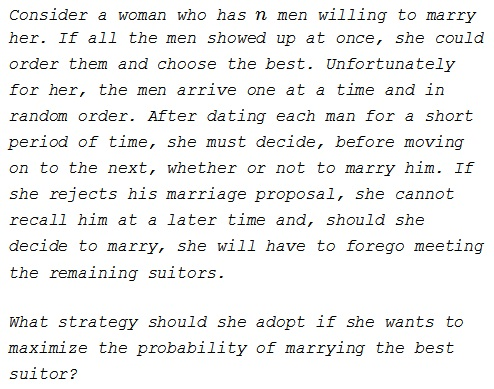# The Marriage Problem

### Problem### Solution 1

Let $X_1,X_2,\ldots,X_n$ be the relative ranks of men so that $X_j$ is the rank of the $j^{th}$ man among the first $j$ men that she dates (with rank $1$ being the best). These random variables are independent, and since all of the arrival orderings are equally likely, $P(X_1 = 1) = 1,$ $\displaystyle P(X_2 = 1) = P(X_2 = 2) = \frac{1}{2},\ldots$ We will call a suitor at stage $j$ a candidate if $X_j = 1.$ Clearly she should reject a suitor who is not a candidate. Note that the probability that the best suitor among the first $j$ is the best overall is just the probability that the best suitor appears among the first $j$ suitors which is $\displaystyle\frac{j}{n}.$ Let $W_j$ represent the maximum probability of selecting the best suitor using a strategy that rejects the first $j$ suitors. Then $W_j\ge W_{j+1}$ since any rule that rejects the first $j + 1$ suitors rejects the first $j$ suitors. Thus it will be optimal to accept a candidate at stage $j$ if $\displaystyle \frac{j}{n}\ge W_j.$ Moreover, since

$\displaystyle \frac{j + 1}{n} \gt \frac{j}{n}\ge W_j\ge W_{j+1},$

our bride-to-be can use a threshold rule, that is, a rule of the form "reject $r-1$ suitors and then accept the next candidate, if any, who is better than all previously appearing suitors." Here $r\ge 1.$ Let $P_r$ be the probability of selecting the best suitor using the threshold rule with threshold $r.$ Then

\displaystyle\begin{align} P_r&=\sum_{k=r}^nP(\text{suitor } k\text{ is best and is selected})\\ &=\sum_{k=r}^nP(\text{suitor } k\text{ is best})P(\text{suitor } k\text{ is selected}|\text{suitor }k\text{ is best})\\ &=\sum_{k=r}^n\frac{1}{n}P(\text{best of the first }k-1\text{ appears before stage }r)\\ &=\sum_{k=r}^n\frac{1}{n}\cdot\frac{r-1}{k-1}=\frac{r-1}{n}\sum_{k=r}^n\frac{1}{k-1}, \end{align}

where the first term in the series is interpreted as $1$ when $r = 1.$ The optimal value of $r$ is the value that maximizes $P_r.$ Note that $P_r\ge P_{r+1}$ if and only if

$\displaystyle\frac{r-1}{n}\sum_{k=r}^n\frac{1}{k-1}\ge\frac{r}{n}\sum_{k=r+1}^n\frac{1}{k-1}$

or, equivalently,

$\displaystyle 1\ge\sum_{k=r+1}^n\frac{1}{k-1}.$

If $\displaystyle f(r) =\sum_{k=r+1}^n\frac{1}{k-1},$ then $f(r)$ is decreasing and, therefore, the optimal value $r^*$ is the first $r$ that satisfies that condition. For large $n,$ $\displaystyle f(r)\approx\ln\left(\frac{n}{r}\right),$ and so $\displaystyle\ln\left(\frac{n}{r^*}\right)\approx 1,$ i.e., $\displaystyle\frac{r^*}{n}\approx e^{-1}.$ Thus it is optimal to reject roughly $\displaystyle\frac{1}{e}$ or $37%$ of the suitors before looking for candidates and the probability of success, $P_r,$ is approximately $\displaystyle\frac{1}{e}.$

### Solution 2

You stand in an office by aa door, with a measuring tape. Every time a person walks in you measure him or her and only keep tally of the "record" tallest. If the new person is taller than a preceding one, you count a record. If later another person is taller, you have another record, etc.

A 1000 persons pass through the door. How many records do you expect to have?

(Assume independence of height/arrival. Also note that the answer does not depend on any assumption about the probability distribution other than independence.)

The number of records expected over $n$ periods starting from $r$, a proportion of $n$ is

$\displaystyle\mathbb{E}(X; r,n) =\sum _{i= \lfloor r n \rfloor}^n \frac{1}{i+1} =H_{n+1}-H_{\lfloor n r\rfloor}$

where $H$ is the harmonic number.

We try to solve for $r:\,\mathbb{E}(X; r,n)=1$ and we get $r\approx\frac{1}{e}$, which becomes exact at the limit:

$\displaystyle\lim_{n\to \infty}\mathbb{E}(X; \frac{1}{e},n)=1$

Meaning go through about a $\frac{1}{e}$ proportion then select the first record after that.

Note: Actually, by another route,

$\displaystyle\underset{n\to \infty }{\text{lim}}\left(H_{n+1}-H_{n r}\right)=-\log (r).$

### Acknowledgment

This problem was invented by Martin Gardner in 1960. Its first solution was found by E. B. Dynkin in 1963 and generalized in 1966 by Sabir Gusein-Zade, at the time a freshman at the Moscow State University.

The above is a variant of Dynkin's proof and comes from an article Probability $1/e$ by Reginald Koo and Martin L. Jones (The College Mathematics Journal, VOL. 42, NO. 1, 2011). The authors note that this problem appeared in An Introduction to Mathematical Statistics and Its Applications by R. J. Larsen, and M. L. Marx (pp 90-95 ,Prentice Hall, 1985) as the "Beauty Pageant Problem" and is also known as the "Classical Secretary Problem," "The Fussy Suitor Problem," and the "Best Choice Problem." The above solution follows Optimal Stopping and Applications by T. S. Ferguson.

Solution 2 is by N. N. Taleb.